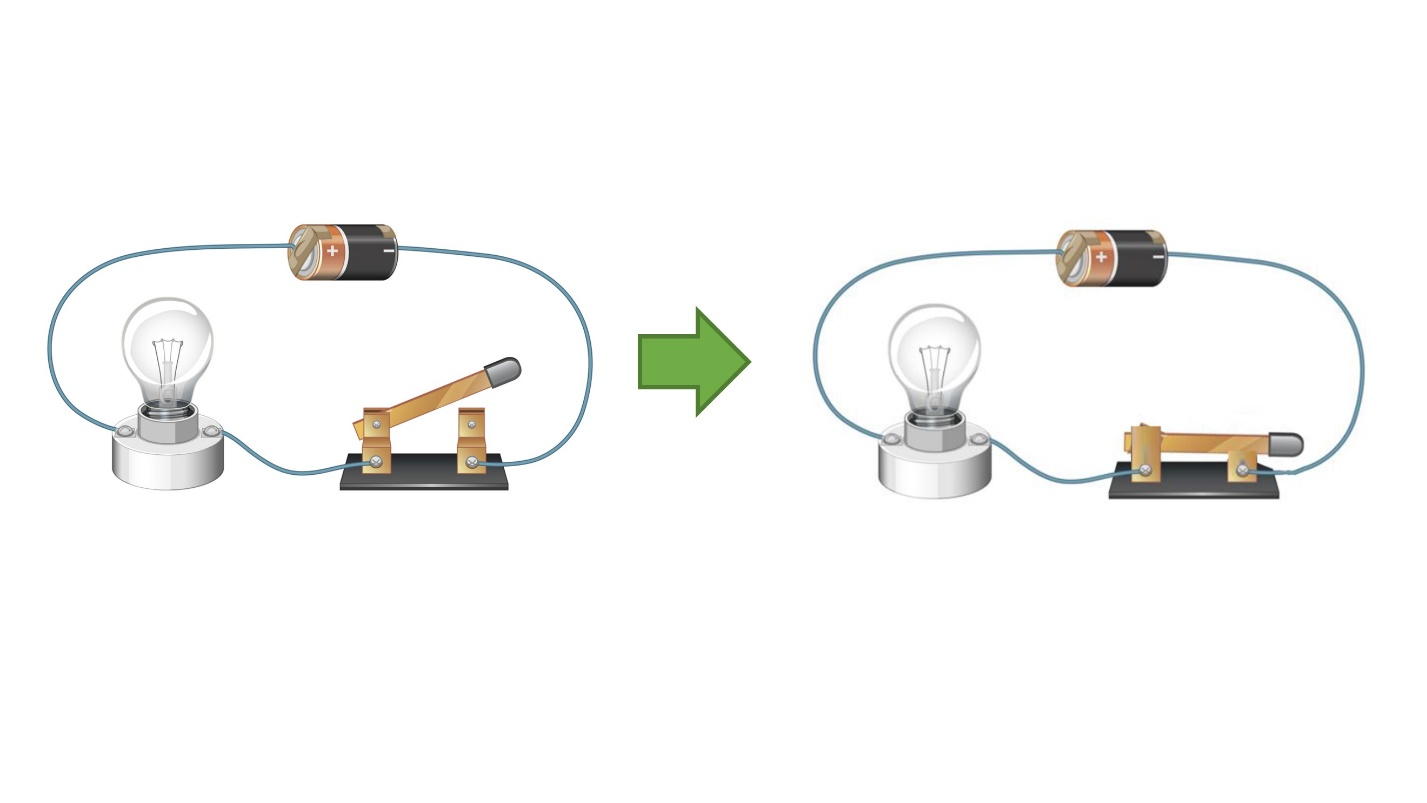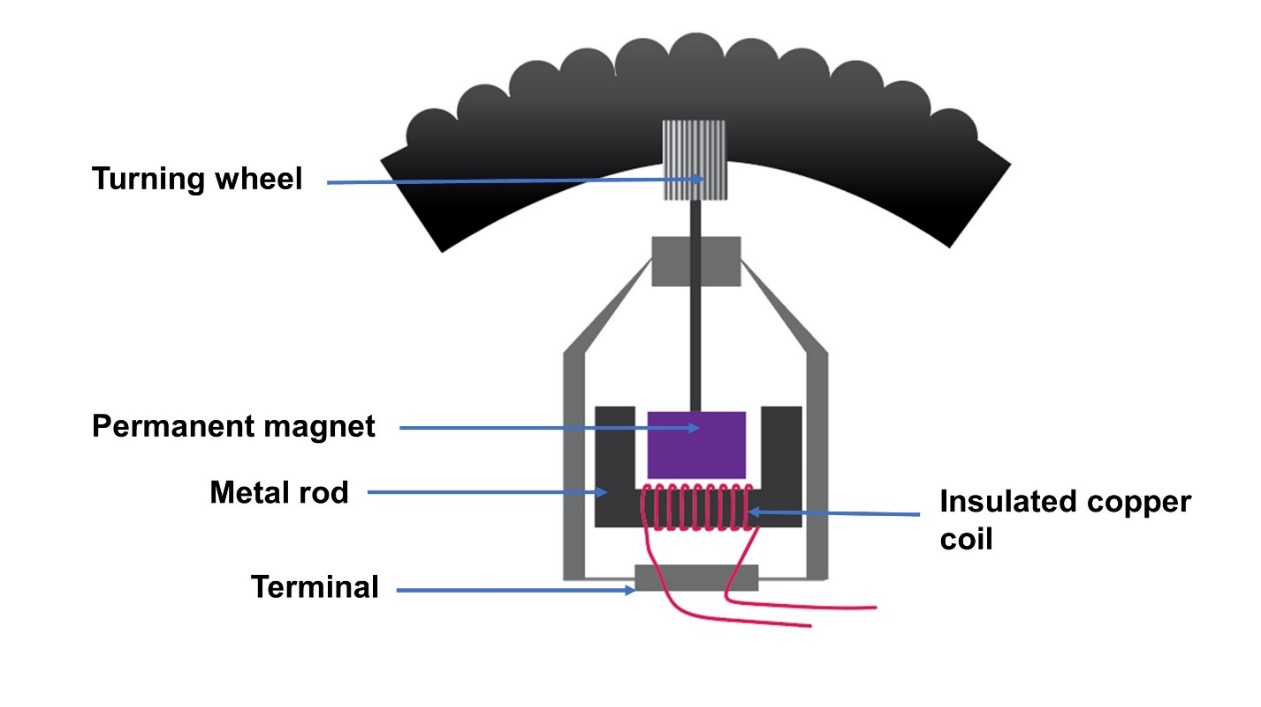## What is an electric current?

The flow of electrical charges through a closed circuit is known as an electric current

## Let us make a circuit

• Let us connect an external wire of an electric source to an electric appliance (bulb).Electric current flows from the source through the wire and the appliance.

### Observations –

The bulb lights up because the appliance starts to work.

## Let’s identify the direction of the current flowing in a circuit.

### Method –

• A dry cell is used as the electrical source.
• Let’s connect this circuit in this way.
• The positive terminal of the dry cell is connected to the motor by the red crocodile pin.
• The negative terminal of the dry cell is connected to the motor by the black crocodile pin.
• Then observe the direction of rotation of the fan in the motor.

### Observations –

The fan getting to rotate.

The direction of the current is indicated by the rotational direction of the fan.

### Conclusion –

It is clear that the electric current flows from the (+) positive terminal to the (-) negative terminal of the source through the circuit

• Change the terminals connected to the circuit and note down the observations. Note down the observations.

When the terminals of the dry cell changed, the direction the motor turns changed as well.

The reason for this is the change of direction of the current.

It is clear that the electric current flows from the (+) positive terminal to the (-) negative terminal of the source through the circuit

A center-zero galvanometer or a center-zero ammeter can be used to identify the direction of an electric current.We use a center-zero galvanometer for this practical.

### Consider;

• The positive and negative terminals of the dry cell should be connected to the positive and negative terminals of the galvanometer respectively.
• The direction of the current is shown by the indicator of the galvanometer.

It is clear that the electric current flows from the (+) positive terminal to the (-) negative terminal of the source through the circuit